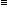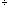Date: Wed, 3 Feb 1999 16:21:27 -0600 (CST)
Subject: modular arithmetic

Name: Leslie

Level: All

Question:
I am trying to do a project on modular arithmetic. I was wondering if there were any websites that include a sample lesson plan on modular arithmetic for any grade level. Let me know where and how to find them. Thanks.

Hi Leslie

What follows is a section from an online course that is offered at the University of Regina. It is not lesson plans but perhaps it will give you some ideas.

Harley

# Arithmetic and Numeration Systems

## Modular Arithmetic

Earlier on in this chapter we asked:
• counting in hours - if it is 10:00 a.m. what time will it be 30 hours from now?
• counting in days - if it is Wednesday what day will it be 452 days from now?
• counting degrees - if you are facing north and spin clockwise 810 degrees, which way are you facing?

The idea that we need to use in these problems is counting in multiples -- of 12's, 7's, and 360's. In the first case we are only interested in the fact that 30 is 6 more than 24 and that 10 (a.m.) plus 6 leaves a remainder of 4 when we take away 12; in the second case that 452 leaves a remainder of 4 when we take away multiples of 7; 810 leaves a remainder of 90 when we take away multiples of 360. Thus we have as solutions: 4 p.m., Sunday and East.

In a more formal manner we say that (for integers a, b and m) that a is congruent to b modulo m if a and b leave the same remainder when divided by m. We usually denote this symbolically by ab (mod m). This idea of congruence was first developed by the mathematician Carl Friedrich Gauss in the late 18th century. In our examples above we have 306 (mod 12); 4524 (mod 7); 81090 (mod 360).

Equivalent ways of stating the relationship of congruence include:

• a leaves a remainder of b when divided by m
For example: 104(mod 6), because 106 has a remainder of 4.
• a - b0 (mod m) i.e. a - b is a multiple of m
For example: 10-2(mod 6), because 10 -(-2) = 12, which is a multiple of 6, and multiples of 6 have no remainders when divided by 6.
• a = tm + b for some integer t.
For example: 394(mod 7), because 39 = (5)(7) + 4.

NOTE: Remember, with congruences we will be restricting ourselves to the integers.

More examples:
 101 (mod 3); 5-3 (mod 4); 10231 (mod 2); 1023-1 (mod 2); 275 (mod 11); -184 (mod 7)

## Exercises

 1. 83__ (mod 2) 4. 78__ (mod 7) 7. 15__ (mod 6) 2. 23__ (mod 5) 5. 95__ (mod 11) 8. 81__ (mod 9) 3. 26__ (mod 12) 6. 16__ (mod 2) 9. 30__ (mod 8)

When we do modular arithmetic we throw away multiples of the modulus. Thus for example if we add 23 and 14 modulo 5 we end up with 37 modulo 5 which is 2 modulo 5; briefly 23 + 14 = 372 (mod 5). Similarly 1629 = 4642 (mod 3). We can shorten our work sometimes by reducing the terms that we are working with by the modulus, thus 23 + 143 + 4 = 72 (mod 5), and 162912 = 2 (mod 3). The salient point here is that we can throw away multiples of the modulus m before the arithmetic operation if we wish.

Let's look at addition and multiplication modulo 5. We arrange the results in tables as follows:

+01234
001234
112340
223401
334012
44012301234
000000
101234
202413
303142
404321

For modulo 6 we have:

+012345
0012345
1123450
2234501
3345012
4450121
5501234012345
0000000
1012345
2024024
3030303
4042042
5054321

Note that in modular arithmetic we can have a times b is 0 even when a and b are both non-zero, for example in (mod 6), 320 (mod 6), quite different from our usual arithmetic!

We can deal with equations in modular arithmetic in a manner very similar to that which we are used to in ordinary arithmetic. For example, solve 3z + 24 (mod 7) asks which integer(s) satisfy the congruence. Before we solve this problem we should observe that the equation 3z - 2 = 7 has the single solution of z = 3. When we deal with congruences many solutions, possibly infinitely many, may occur. Why is this? Well, consider 3z + 24 (mod 7) or 3z - 20 (mod 7). z = 3 is a solution since 33 - 2 = 70 (mod 7) but notice also that 10 also works for 310 - 2 = 280 (mod 7). In fact once we have found the solution of z = 3 we have infinitely many solutions {3, 3 ± 7, 3 ± 27, 3 ± 37, 3 ± 47, ..., 3 ± t7, ... } (which is the same as the set of number {..., -25, -18, -11, -4 , 3, 10, 17, 24, ...}) since adding (or subtracting) multiples of 7 to our solution gives new solutions modulo 7: for example 3( 3 + 527) - 233 - 2 = 70 (mod 7). An important idea is buried in all of this -- when solving congruences we need only find one solution to find them all! We simply then add multiples of the modulus to find other solutions. Even more, this allows us to restrict our search, in the mod 7 case, for the first solution to the number 0, 1, 2, ..., 6.

Examples:

Solve 4z + 12 (mod 9).
Let's rewrite this as solve 4z - 10 (mod 9). Now try z = 0, 1, 2, ..., 8: for these values of z we have that 4z - 1 = -1, 3, 7, 11, 15, 19, 23, 27, 31 respectively; of these we want only multiples of 9, namely when z = 7, thus Solve 4z + 12 (mod 9) has as solutions the set of numbers {7, 7 ± 9, 7 ± 18, 7 ± 27,...} = {..., -20, -11, -2, 7, 16, 25, 34,...}.

Solve 5z - 13 (mod 8).
Let's rewrite this as solve 5z - 40 (mod 8). Now try z = 0, 1, 2, ..., 7: for these values of z we have that 5z - 4 = -4, 1, 6, 11, 16, 21, 26, 31; we see that we want z = 4, thus solve 5z - 13 (mod 8) has solutions, {4, 4 ± 8, 4 ± 16,...} = {..., -12, -4, 4, 12, 20,...}.

Solve 2z1 (mod 4), equivalently, 2z - 10 (mod 4).
We try z = 0, 1, 2, 3, and find 2z - 1 = -1, 1, 3, 5, none of which are multiples of 4 so 2z1 (mod 4) has no solutions. In other words, 2z - 1 can not be congruent to 0 (mod 4).

## Exercises

1. Construct the addition and multiplication tables for mod 7 arithmetic.

2. Find the smallest positive solution to
 2. 3456x (mod 11) 3. 823234z (mod 8)

3. Find all solutions, if any, to
 4. 7x3 (mod 11) 5. 4z1 (mod 6)
Go to Math Central